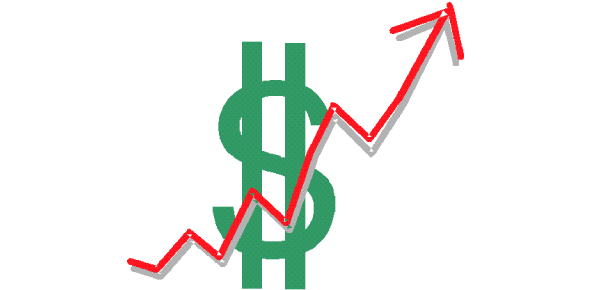# Economics Trivia MCQ: Exam! Quiz

30 Questions | Total Attempts: 317Settings.

• 1.
GDP per capita is GDP divided by:
• A.

GNP

• B.

The size of the population

• C.

The size of the labor force

• D.

Twenty-five

• 2.
"The total of all economic activity in a country" What is being defined?
• A.

GNP

• B.

GDP

• C.

Nominal GDP

• D.

Deflationary gap

• 3.
Fiscal policy is the set of a government's policies relating to its ...
• A.

Money supply

• B.

Money supply and control of interest rates

• C.

Control of interest rates and taxation rates

• D.

Spending and taxation rates

• 4.
"The periodic fluctuations in economic activity measured by changes in real GDP" What is being defined?
• A.

Depreciation

• B.

Multiplier effect

• C.

• D.

Accelerator theory

• 5.
Diffrence between Real and Nominal GDP is:
• A.

Measured by excluding some of the sectors

• B.

That real GDP is always smaller than Nominal GDP.

• C.

Change in price level from base year to current year.

• D.

None of the above.

• 6.
The fiscal policy of 2077/78 of Nepal targets inflation at:
• A.

7%

• B.

5.5%

• C.

6.5%

• D.

6%

• 7.
Which is not the component of the current account under Balance of Payment?
• A.

• B.

Remittance

• C.

Interest repatriation

• D.

Greenfield investment

• 8.
The CCD ratio for BFIs in Nepal stands at:
• A.

80 percent

• B.

75 percent

• C.

85 percent

• D.

90 percent

• 9.
Which is the only European country to remain unaffected by the great depression of the 1930s?
• A.

Russia

• B.

Germany

• C.

France

• D.

Britain

• 10.
Which of the following statement is not true?
• A.

The inside lag of fiscal policy is longer than that of monetary policy

• B.

The outside lag of fiscal policy is shorter than that of monetary policy

• C.

The monetary policy has significantly faster impact on economic variables

• D.

Fiscal policy takes longer time to formulate

• 11.
The real sector of the Nepalese economy is divided into how many sectors?
• A.

10

• B.

15

• C.

20

• D.

12

• 12.
World Bank has said that the environment for doing business in Nepal has improved. What is the ranking of Nepal?
• A.

94

• B.

105

• C.

90

• D.

106

• 13.
Which is not the instrument of monetary policy?
• A.

CCD ratio

• B.

Open Market Operation

• C.

Bank rate

• D.

SLR

• 14.
Which of the following increases the real GDP?
• A.

Increase in real interest rate

• B.

Increase in gross investment

• C.

Increase in final consumption

• D.

None of above

• 15.
A change in fiscal policy affects the balance of payment through:
• A.

Current account

• B.

Capital account

• C.

Current and capital account

• D.

None of above

• 16.
What is the expected nominal GDP (Rs.) of Nepal for 2019/20 estimated by Central Bureau of Statistics?
• A.

37.67 billion

• B.

34.58 billion

• C.

36.78 billion

• D.

38.45 billion

• 17.
The annual population growth rate of Nepal stands at:
• A.

1.35%

• B.

2%

• C.

2.15%

• D.

1.25%

• 18.
The average revenue of the firm is represented by:
• A.

Demand curve

• B.

Supply curve

• C.

Marginal revenue

• D.

None of above

• 19.
Imposing taxes to which of the following goods yield the highest revenue to government?
• A.

Alcoholic drinks

• B.

Vehicles

• C.

Consumables

• D.

All of above

• 20.
In which condition, Seller can shift entire burden of tax to buyer
• A.

If supply curve is perfectly elastic assuming demand constant

• B.

If demand curve is perfectly elastic assuming supply constant

• C.

If both demand and supply curve are relatively elastic

• D.

None of above

• 21.
The tradition Philips curve states that unemployment and inflation move in
• A.

Opposite direction

• B.

Same direction

• C.

They are not related to each other

• D.

None of above

• 22.
Stagflation is best explained by which of the following statement?
• A.

Nepalese economy is facing price surge and a decrease in employment rate

• B.

Nepalese economy is struggling with deflationary pressures and a rapid rise in unemployment rate.

• C.

Nepalese economy is experiencing a huge fiscal deficit and deficit in balance of payment.

• D.

None of above

• 23.
The first five year plan of Nepal started in which of the following fiscal year (BS)?
• A.

1955/56

• B.

1956/57

• C.

1954/55

• D.

1959/60

• 24.
The poverty reduction strategy paper was introduced in which periodic plan?
• A.

5th

• B.

6th

• C.

7th

• D.

8th

• 25.
How many National Pride Projects are currently recognized by 46th National Development Action Committee report?
• A.

22

• B.

21

• C.

23

• D.

24

Related TopicsBack to top